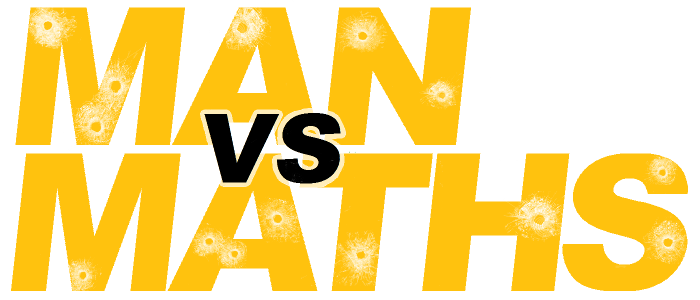# Year 10 Revision

## Practice Exams

Here are two exams that are the same length and difficulty as the final exams, with answers for each of the five sections: Algebra, Graphs, Measurement, Number and Geometry.

Practice Exam #1     Answers Exam #1

Practice Exam #2     Answers Exam #2

## Number Theory (Number I)

Number Theory Notes

Most of this should be quite familiar, but there are a few extra topics, such as Scientific Notation.

Number Terms Cards

The terms and concepts you need to know. These are set up to be printed as flash cards.

Practice 1     Practice 2     Practice 3

Twenty mixed questions on one sheet, with answers on a second sheet.

## Percentages, Fractions, Ratios and Proportion (Number II)

Percentages etc Notes

Notes on percentages, fractions, ratios and proportion.

Practice 1     Practice 2     Practice 3

Twenty mixed questions on one sheet, with answers on a second sheet.

## Measurement

Measurement Notes

Notes on all the key skills.

### Units, Conversions, Errors

Practice 1     Practice 2     Practice 3

Twenty routine questions on one sheet, with answers on a second sheet.

### Calculating Perimeter, Area and Volume

Practice 1     Practice 2     Practice 3

Practice 4     Practice 5     Practice 6

Twelve routine questions on one sheet, with answers on a second sheet.

Extension 1     Extension 2     Extension 3

Some harder shapes to calculate, with a bit of work on estimation and limits of accuracy.

## Algebra

Algebra Notes

What you should be able to do.

Most skills are also available in video form on the Videos Page. Worksheets are available on the Algebra Page.

## Patterns and Graphs (Algebra II)

Patterns and Graphing Notes

What you should be able to do, with some extension.

This allows students to test their ability to draw lines and parabolas from formulas and to write equations from drawn lines and patterns.

Practice 1     Practice 2     Practice 3

Straight line questions, with answers on a second sheet.

Extension 1     Extension 2     Extension 3

These sheets are preparation for Excellence students for Year 11. They have work on parabolas, more difficult patterns and different ways of writing straight lines that is not required for most Year 10 students.

## Shapes and Angles (Geometry I)

Geometry Cards

Terms and concepts you need to know. These are set up to be printed as flash cards.

Practice 1     Practice 2     Practice 3

Geometry questions, with answers on a second sheet.

## Trigonometry (Geometry II)

Trigonometry I     Trigonometry II

The basic notes in part I, with all the skills required. Extension material in part II.

Practice 1     Practice 2     Practice 3

Routine questions, with answers on a second sheet.

Extension 1     Extension 2     Extension 3

Extension trigonometry questions, with answers on a second sheet.

## Transformations (Geometry III)

Transformations Cards

The terms and concepts you need to know. These are set up to be printed as flash cards.

Reflection 1     Rotation 1     Translation 1

Reflection 2     Rotation 2     Translation 2

Enlargement 1     Isometric 1

Enlargement 2     Symmetry 1

Revison of all Transformations

Worksheets on the various parts of the topic, with answers on a second sheet.

## Statistics

Statistics Notes

The scope of the whole topic, in quite a lot of detail.

Statistics Quick Reference Sheet

A page on the way to approach answering the question in the test.

Statistics Cards

Terms and concepts you need to know. These are set up to be printed as flash cards.

Practice 1     Practice 2     Practice 3

Statistics questions using a range of various graphs and techniques. Suggested answers are on the second page.

Practice 4     Practice 5     Practice 6

Each worksheet is two box-and-whisker interpretation questions (the first easy, the second a bit harder). The answers are only a suggestion in these worksheets, because not every possible correct answer can be provided and students don't have to mention every detail. Students can bring them to me to mark if they prefer.

Extension 1     Extension 2     Extension 3

These exercises are practice for the Level 1 assessments. Each is two pages (consisting of the questions, data and graph paper) and then two pages of model answers. Students can bring them to me to mark, if they wish.

## Probability

Probability Notes

The basic concepts for this year.

Practice 1     Practice 2     Practice 3

Probability questions, with answers on a second sheet.